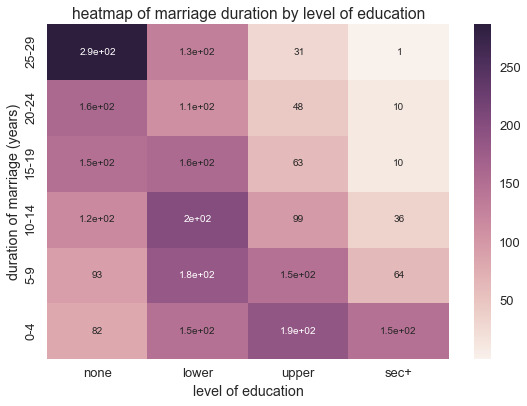# Count Data and Ordinal Data with the Gamma-Poisson Distribution¶

Typically, we model count data, or integer valued data, with the gamma-Poisson distribution

Recall that the Poisson distribution is a distribution over integer values parameterized by $$\lambda$$. One interpretation behind $$\lambda$$ is that it parameterizes the rate at which events occur with a fixed interval, assuming these events occur independently. The gamma distribution is conjugate to the Poisson distribution, so the gamma-Poisson distribution allows us to learn both the distribution over counts and the rate parameter $$\lambda$$.

Let’s set up our environment and consider some examples of count data

import pandas as pd
import seaborn as sns
import numpy as np
import matplotlib.pyplot as plt
sns.set_context('talk')
import csv
import urllib2
import StringIO

%matplotlib inline


Children Ever Born is a dataset of birthrates in Fiji from the World Fertility Survey with the following columns:

• dur: marriage duration
• res: residence,
• educ: level of education,
• mean: mean number of born,
• var: variance of children born
• y: number of women

Ordinal columns dur, res, and educ are shown as text in the following dataset

ceb = pd.read_csv('http://data.princeton.edu/wws509/datasets/ceb.dat', sep='\s+')

dur res educ mean var n y
1 0-4 Suva none 0.50 1.14 8 4.00
2 0-4 Suva lower 1.14 0.73 21 23.94
3 0-4 Suva upper 0.90 0.67 42 37.80
4 0-4 Suva sec+ 0.73 0.48 51 37.23
5 0-4 urban none 1.17 1.06 12 14.04

With the these columns encoded, we can now represent them as integers

dur and educ are ordinal columns. Additionally, number of women, n, is integer valued.

ceb_int = pd.read_csv('http://data.princeton.edu/wws509/datasets/ceb.raw', sep='\s+', names = ['index'] + list(ceb.columns[:-1]), index_col=0)

dur res educ mean var n
index
1 1 1 1 0.50 1.14 8
2 1 1 2 1.14 0.73 21
3 1 1 3 0.90 0.67 42
4 1 1 4 0.73 0.48 51
5 1 2 1 1.17 1.06 12

We can map these orderings of dur and educ to produce a crosstab heatmap of n, numbe of women

plt.figure(figsize=(9,6))
ct = pd.crosstab(ceb_int['dur'], ceb_int['educ'], values=ceb_int['n'], aggfunc= np.sum).sort_index(ascending = False)
sns.heatmap(ct, annot = True)
plt.yticks(ceb_int['dur'].drop_duplicates().values - .5, ceb['dur'].drop_duplicates().values)
plt.xticks(ceb_int['educ'].drop_duplicates().values - .5, ceb['educ'].drop_duplicates().values)
plt.ylabel('duration of marriage (years)')
plt.xlabel('level of education')
plt.title('heatmap of marriage duration by level of education')

<matplotlib.text.Text at 0x11a8f48d0>Since dur and education are ordinal valued, the columns assume a small number of integer values

Additionally, the caffeine dataset below measures caffeine intake and performance on a 10 question quiz. The variables are:

• coffee: coffee intake (1 = 0 cups, 2 = 2 cups, 3 = 4 cups)
• perf: quiz score
• numprob: problems attempted
• accur: accuracy
response = urllib2.urlopen('http://stanford.edu/class/psych252/_downloads/caffeine.csv')

coffee perf accur numprob
0 1 53 0.449877 7
1 1 9 0.499534 6
2 1 31 0.498590 6
3 1 38 0.454312 7
4 2 40 0.421212 8

Based on the characteristics of each column, coffee and numprob easily fit into the category of count data appropriate to a gamma-Poisson distribution

caf.describe()

coffee perf accur numprob
count 60.000000 60.000000 60.000000 60.000000
mean 2.000000 42.366667 0.510854 7.950000
std 0.823387 18.350603 0.107704 1.185005
min 1.000000 5.000000 0.240238 6.000000
25% 1.000000 31.000000 0.425859 7.000000
50% 2.000000 40.000000 0.509806 8.000000
75% 3.000000 53.500000 0.594445 9.000000
max 3.000000 89.000000 0.748692 10.000000

Note that while integer valued data with high values is sometimes modeled with a gamma-Poisson ditribution, remember that the gamma-Poisson distribution has equal mean and variance $$\lambda$$:

$E(X) = Var(X) = \lambda$

If you want to be more flexible with this assumption, you may want to consider using a normal inverse-chisquare or a normal inverse-Wishart distribution depending on your data

To import the gamma-poisson likelihood, call:

from microscopes.models import gp as gamma_poisson